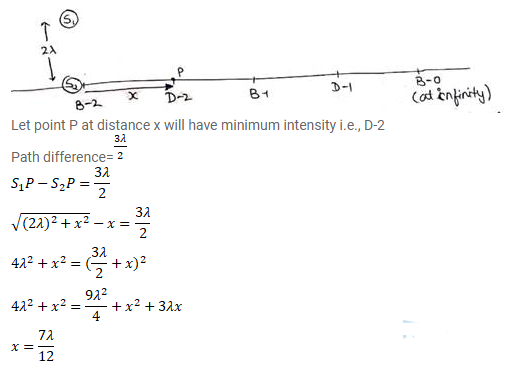# Two coherent point sources S1 and S2 vibrating in phase emit light of wavelength λ.Question:

Two coherent point sources $S_{1}$ and $S_{2}$ vibrating in phase emit light of wavelength $\lambda$. The separation between the sources is 2 $\lambda$. Consider a line passing through $S_{2}$ and perpendicular to the line $S_{1}, S_{2}$. What is the smallest distance from $S_{2}$ where a minimum of intensity occurs?

Solution: